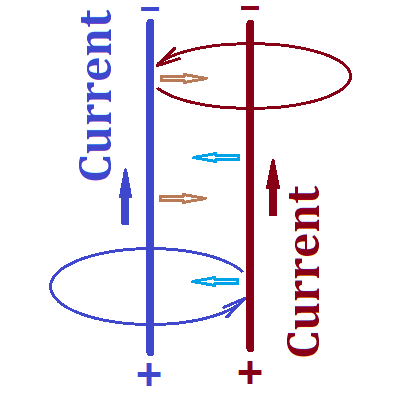## Wednesday, June 3, 2020

### Unizor - Physics4Teens - Electromagnetism - Magnetism and Electric Curre...

Notes to a video lecture on http://www.unizor.com

Two Parallel Straight Line Currents

Let's examine a behavior of two ideally long and thin parallel wires, each carrying an electric current.Two wires carrying an electric current are blue and brown on this picture.

As we know, since the wires carry electric current, there is a magnetic
field around each of these wires. It has circular form, as presented on a
picture above in corresponding colors. The direction of the magnetic
field lines is determined by the corkscrew rule or the right hand rule and is shown on the picture.

Each circular magnetic field line around the blue wire and any
tangential to it, that is the vector of magnetic force, belong to a
plane perpendicular to this wire. Since the brown wire is parallel to
the blue one, any tangential to any magnetic field line of the blue wire
is perpendicular to the brown wire.

Since the brown wire's electric current is perpendicular to the
direction of the magnetic field lines of the field produced by the blue
wire, the brown wire is under the Lorentz force produced by the magnetic
field of the blue wire. Using the right hand rule for the brown
wire and blue magnetic field lines, we determine the direction of this
Lorentz force. It appears to be directed from the brown wire towards the
blue one.

The wires are in symmetrical positions, so exactly the same logic can be
applied to the blue wire in the magnetic field of the brown wire. As a
result, the Lorentz force produced by the magnetic field of the brown
wire attracts the blue wire towards the brown one.

Now we see that both wires attract each other, which is caused by the
Lorentz force exerted on each of them from the magnetic field of the
other one.

Let's arrange a similar experiment having electric currents in the wires
to go in opposite directions. Let the blue wire's current to go the
same way as on the picture above, that is upward, but the direction of
the electric current in the brown wire to be downward.

The logic about circular magnetic field lines around any wire and
perpendicularity of the electric current of one wire to the magnetic
field lines of the opposite one remains the same. The only difference is
that, when we apply the right hand rule to determine the
direction of the Lorentz force, the result will be opposite than before,
the Lorentz force will push the brown wire away from the blue one.

Analogous result will be when we consider the electric current in the
blue wire in the magnetic field of the brown wire. The Lorentz force in
this case will also be repelling.

The conclusion of these experiments is that

(a) parallel wires carrying electric charge will attract each other if the currents are in the same direction;

(b) parallel wires carrying electric charge will repel each other if the currents are in the opposite directions.

This effect was studied by Ampere and the mutual attraction or repulsion
of two parallel wires carrying an electric current is often referred to
as the Ampere force.

Contemporary definition of an 1 ampere (1A) as a unit of electric current is 1 coulomb per 1 second (1C/1sec) with a unit of charge 1 coulomb defined based on the charge of certain number of electrons.

At the time of Ampere's experiments with parallel wires scientists did
not know about electrons and could not measure the electric current in
the same terms as we do now.

But Ampere's experiments have opened the way to measure the electric
current by measuring the purely mechanical characteristic of the force
of attracting or repelling between the wires carrying the electric
current. So, the first definition of a unit of current was based on
measuring the Ampere force between the two wires.

Here is a simple description of how the electric current can be measured (not necessarily the method used by Ampere).

Let two identical wires hang horizontally parallel to each other on threads of the same length from the same height.

Without an electric current running through them these wires hang on vertical threads.

When we apply voltage to both of them from the same battery, the
electric current in each of them produces the magnetic field that will
cause their mutual attraction or repelling, and the threads holding the
wires would deviate from their vertical position. The angle of deviation
can be measured and it can be used to determine the force of attraction
or repelling between the wires.

So, knowing the unit of length and the unit of force, 1 ampere (1A),
as a unit of electric current, can be defined as the electric current
that produces a unit of force per unit of length of a wire, when this
wire is positioned at a unit of length distance from another wire with
the same electric current.

That was the original method of definition of the unit of electrical
current. At the time it was using old units of length and force.

Later on the definition was slightly changed using concepts of
infinitely long and infinitesimally thin wires (to avoid irregularities
of the magnetic field) and contemporary measures of length (meter) and force (newton).